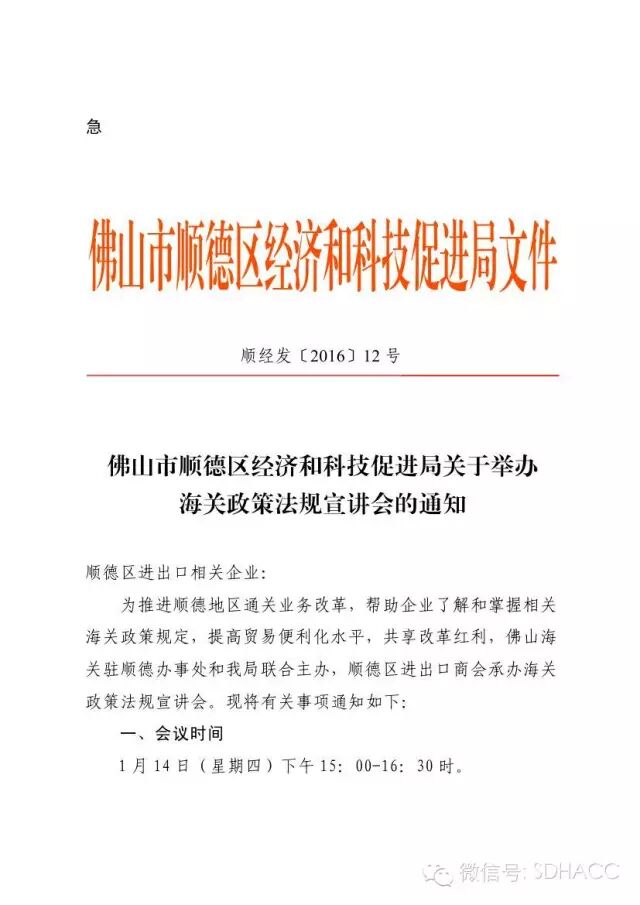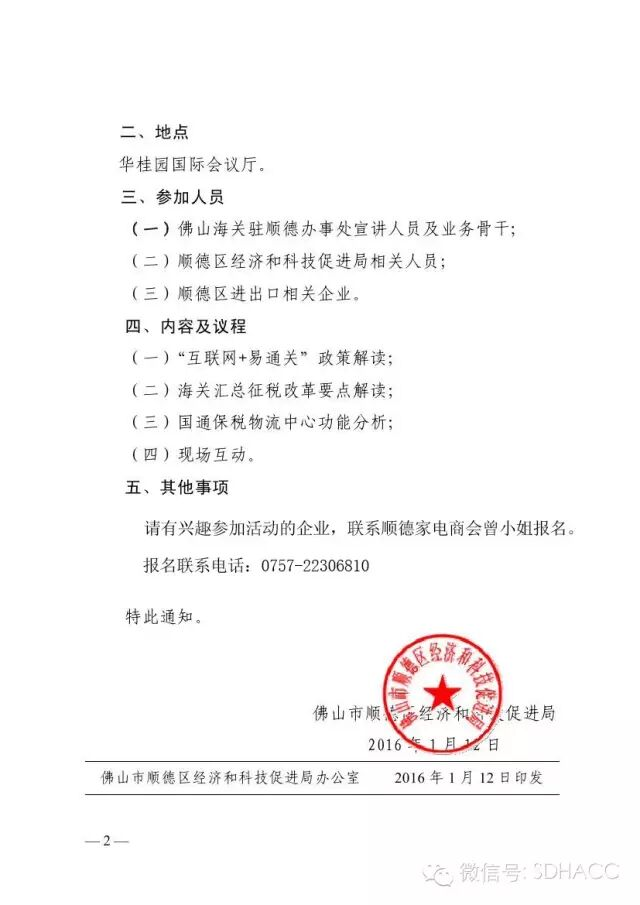## 商会工作 会员动态 资讯中心 供求信息 培训交流 function searchnew(n,m) { var sUrl; var a; var sel=document.getElementsByName("cate_idser"); var p= sel.options[sel.options.selectedIndex].value;//你要的值 if(p=='1'){a = "/index.php?m=Index&c=Commerce&a=index&k={keyword}";} if(p=='2'){a = "/index.php?m=Index&c=Dynamic&a=index&k={keyword}";} if(p=='3'){a = "/index.php?m=Index&c=News&a=index&k={keyword}";} if(p=='4'){a = "/index.php?m=Index&c=Supply&a=index&k={keyword}";} if(p=='5'){a = "/index.php?m=Index&c=Promote&a=index&k={keyword}";} s = encodeURIComponent(document.getElementById(n).value); sUrl = a.replace(/\{keyword}/, s) window.location.href = sUrl; return; }

【2016.01.14 顺德华桂园】区海关政策法规宣讲会
2016-01-22 10:38:57﻿